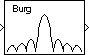# Burg Method

Power spectral density estimate using Burg method

•Libraries:
DSP System Toolbox / Estimation / Power Spectrum Estimation

## Description

The Burg Method block estimates the power spectral density (PSD) of the input frame using the Burg method. This method fits an autoregressive (AR) model to the signal by minimizing (least squares) the forward and backward prediction errors. The block minimizes the errors by constraining the AR parameters to satisfy the Levinson-Durbin recursion.

The block computes the spectrum from the FFT of the estimated AR model parameters.

## Ports

### Input

expand all

Specify the input as a column vector or an unoriented vector. This input represents a frame of consecutive time samples from a single-channel signal.

Data Types: `single` | `double`

### Output

expand all

Power spectral density estimate of the signal at Nfft equally spaced frequency points, returned as a column vector. The frequency points are in the range [0,Fs), where Fs is the sampling rate of the signal.

Data Types: `single` | `double`

## Parameters

expand all

When you select the Inherit estimation order from input dimensions parameter, the order of the all-pole model (estimation order) is one less than the input frame size. Otherwise, the Estimation order parameter determines the model order. The block computes the spectrum from the FFT of the estimated AR model parameters.

Specify the estimation order of the AR model as a nonnegative integer.

#### Dependencies

To enable this parameter, clear the Inherit estimation order from input dimensions parameter.

When you select this parameter, the FFT length Nfft is one greater than the estimation order. To specify the number of points on which to perform the FFT, clear the Inherit FFT length from estimation order parameter. You can then specify the FFT length as a power of 2 using the FFT length parameter. The block zero-pads or wraps the input to Nfft before computing the FFT.

Enter the number of data points Nfft on which to perform the FFT as a positive integer greater than or equal to 2. When Nfft is larger than the input frame size, the block zero-pads each frame as needed. When Nfft is smaller than the input frame size, the block wraps each frame as needed.

#### Dependencies

To enable this parameter, clear the Inherit FFT length from input dimensions parameter.

When you select the Inherit sample time from input parameter, the block computes the frequency data from the sample period of the input signal. For the block to produce a valid output, the following conditions must hold:

• The input to the block is an original signal with no samples added or deleted (by insertion of zeros, for example).

• The sample period of the time-domain signal in the simulation equals the sample period of the original time series.

If these conditions do not hold, clear the Inherit sample time from input parameter. You can then specify a sample time using the Sample time of original time series parameter.

Specify the sample time of the original time-domain signal as a positive scalar.

#### Dependencies

To enable this parameter, clear the Inherit sample time from input parameter.

## Block Characteristics

 Data Types `double` | `single` Multidimensional Signals `No` Variable-Size Signals `No`